Скачать презентацию Text Classification The Problem Statistical text categorization Types

4d46e95fabadcb2da29a0161787317ea.ppt

• Количество слайдов: 18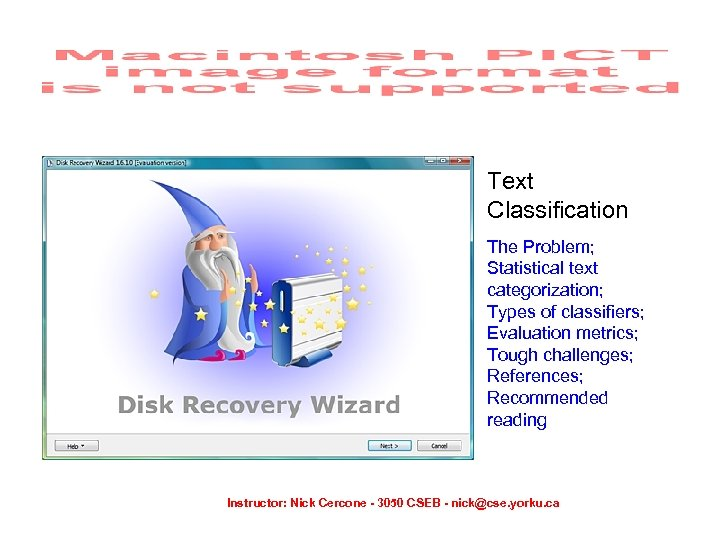Text Classification The Problem; Statistical text categorization; Types of classifiers; Evaluation metrics; Tough challenges; References; Recommended reading Instructor: Nick Cercone - 3050 CSEB - [email protected] yorku. ca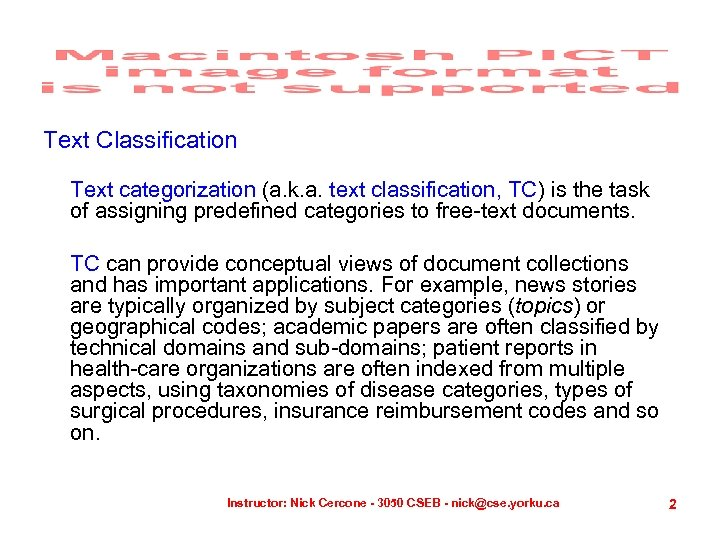Text Classification Text categorization (a. k. a. text classification, TC) is the task of assigning predefined categories to free-text documents. TC can provide conceptual views of document collections and has important applications. For example, news stories are typically organized by subject categories (topics) or geographical codes; academic papers are often classified by technical domains and sub-domains; patient reports in health-care organizations are often indexed from multiple aspects, using taxonomies of disease categories, types of surgical procedures, insurance reimbursement codes and so on. Instructor: Nick Cercone - 3050 CSEB - [email protected] yorku. ca 2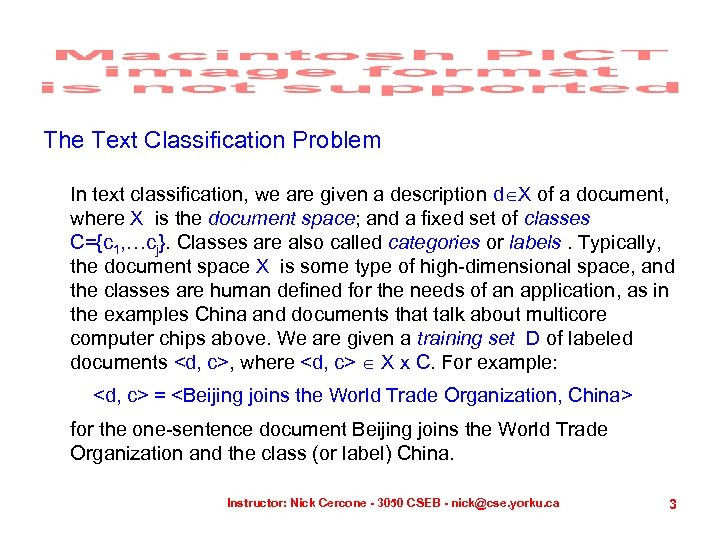The Text Classification Problem In text classification, we are given a description d X of a document, where X is the document space; and a fixed set of classes C={c 1, …cj}. Classes are also called categories or labels. Typically, the document space X is some type of high-dimensional space, and the classes are human defined for the needs of an application, as in the examples China and documents that talk about multicore computer chips above. We are given a training set D of labeled documents , where X x C. For example: = for the one-sentence document Beijing joins the World Trade Organization and the class (or label) China. Instructor: Nick Cercone - 3050 CSEB - [email protected] yorku. ca 3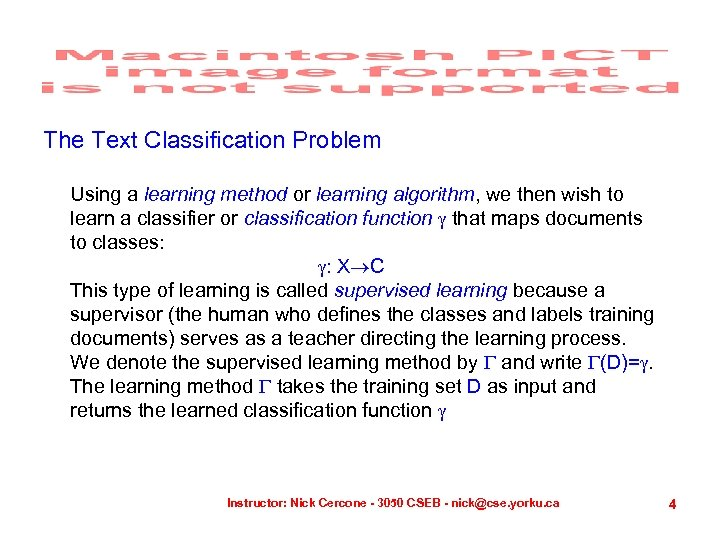The Text Classification Problem Using a learning method or learning algorithm, we then wish to learn a classifier or classification function that maps documents to classes: : X C This type of learning is called supervised learning because a supervisor (the human who defines the classes and labels training documents) serves as a teacher directing the learning process. We denote the supervised learning method by and write (D)=. The learning method takes the training set D as input and returns the learned classification function Instructor: Nick Cercone - 3050 CSEB - [email protected] yorku. ca 4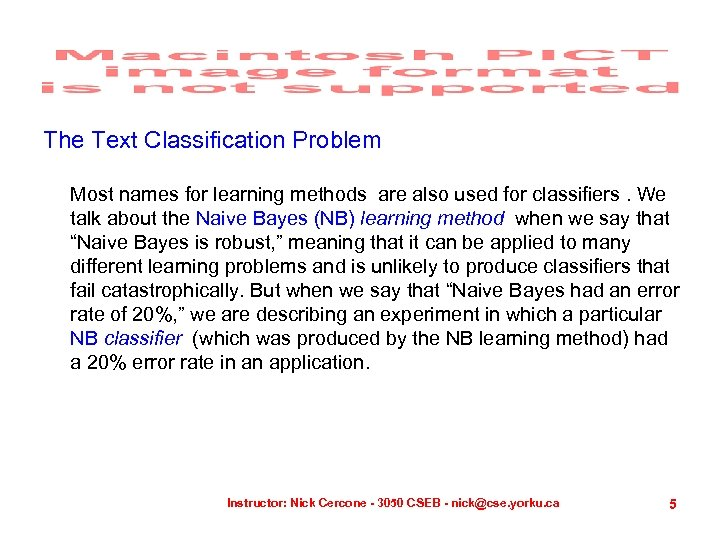The Text Classification Problem Most names for learning methods are also used for classifiers. We talk about the Naive Bayes (NB) learning method when we say that “Naive Bayes is robust, ” meaning that it can be applied to many different learning problems and is unlikely to produce classifiers that fail catastrophically. But when we say that “Naive Bayes had an error rate of 20%, ” we are describing an experiment in which a particular NB classifier (which was produced by the NB learning method) had a 20% error rate in an application. Instructor: Nick Cercone - 3050 CSEB - [email protected] yorku. ca 5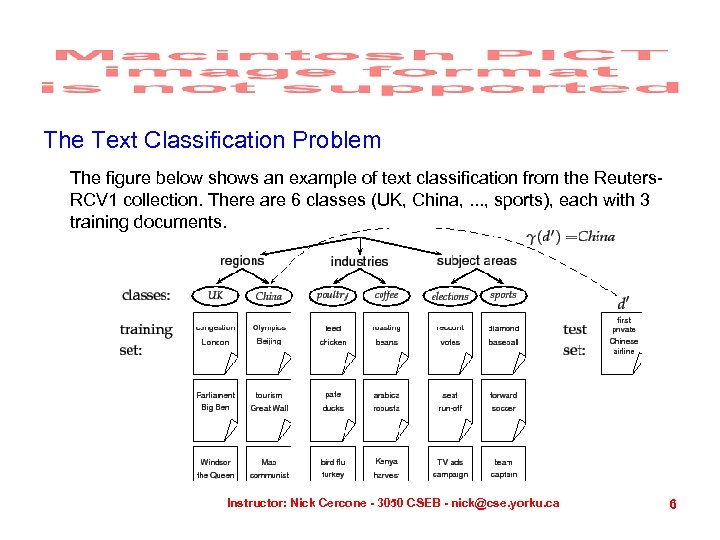The Text Classification Problem The figure below shows an example of text classification from the Reuters. RCV 1 collection. There are 6 classes (UK, China, . . . , sports), each with 3 training documents. Instructor: Nick Cercone - 3050 CSEB - [email protected] yorku. ca 6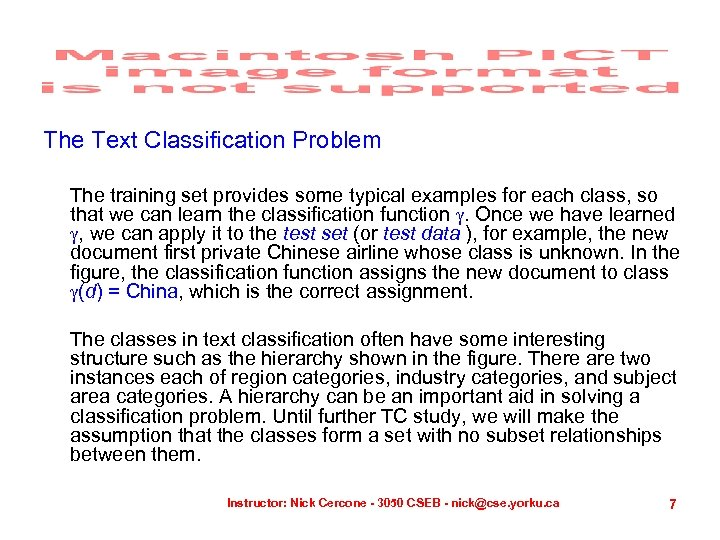The Text Classification Problem The training set provides some typical examples for each class, so that we can learn the classification function . Once we have learned , we can apply it to the test set (or test data ), for example, the new document first private Chinese airline whose class is unknown. In the figure, the classification function assigns the new document to class (d) = China, which is the correct assignment. The classes in text classification often have some interesting structure such as the hierarchy shown in the figure. There are two instances each of region categories, industry categories, and subject area categories. A hierarchy can be an important aid in solving a classification problem. Until further TC study, we will make the assumption that the classes form a set with no subset relationships between them. Instructor: Nick Cercone - 3050 CSEB - [email protected] yorku. ca 7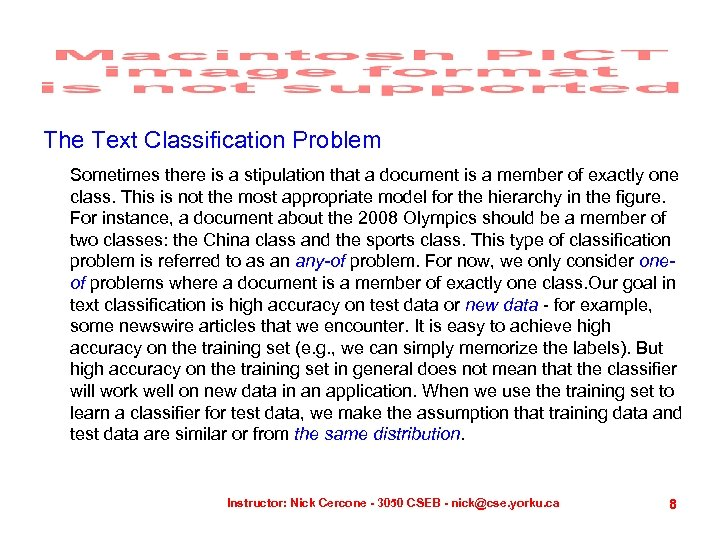The Text Classification Problem Sometimes there is a stipulation that a document is a member of exactly one class. This is not the most appropriate model for the hierarchy in the figure. For instance, a document about the 2008 Olympics should be a member of two classes: the China class and the sports class. This type of classification problem is referred to as an any-of problem. For now, we only consider oneof problems where a document is a member of exactly one class. Our goal in text classification is high accuracy on test data or new data - for example, some newswire articles that we encounter. It is easy to achieve high accuracy on the training set (e. g. , we can simply memorize the labels). But high accuracy on the training set in general does not mean that the classifier will work well on new data in an application. When we use the training set to learn a classifier for test data, we make the assumption that training data and test data are similar or from the same distribution. Instructor: Nick Cercone - 3050 CSEB - [email protected] yorku. ca 8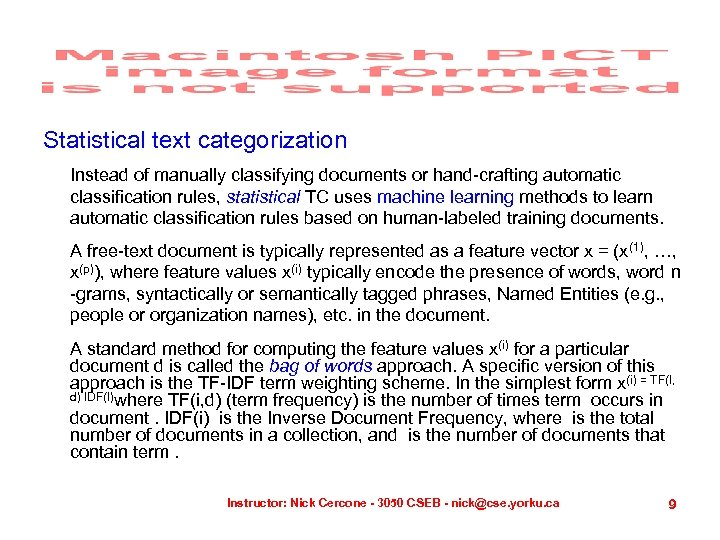Statistical text categorization Instead of manually classifying documents or hand-crafting automatic classification rules, statistical TC uses machine learning methods to learn automatic classification rules based on human-labeled training documents. A free-text document is typically represented as a feature vector x = (x(1), …, x(p)), where feature values x(i) typically encode the presence of words, word n -grams, syntactically or semantically tagged phrases, Named Entities (e. g. , people or organization names), etc. in the document. A standard method for computing the feature values x(i) for a particular document d is called the bag of words approach. A specific version of this approach is the TF-IDF term weighting scheme. In the simplest form x(i) = TF(I, d) IDF(I)where TF(i, d) (term frequency) is the number of times term occurs in document. IDF(i) is the Inverse Document Frequency, where is the total number of documents in a collection, and is the number of documents that contain term. Instructor: Nick Cercone - 3050 CSEB - [email protected] yorku. ca 9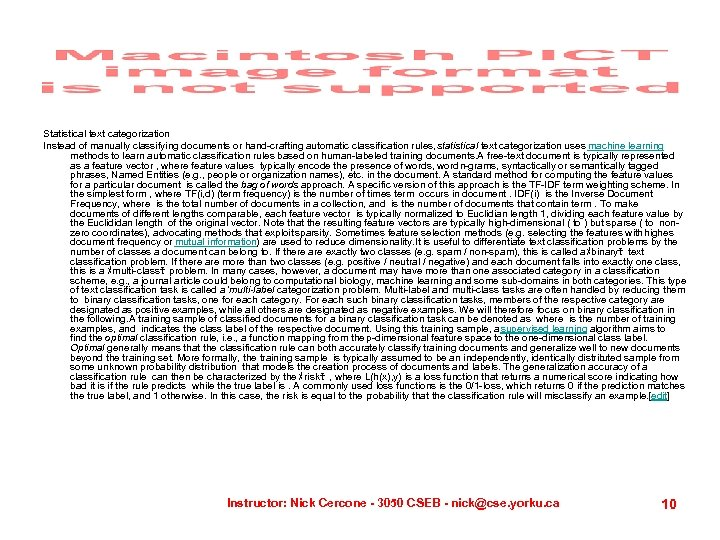Statistical text categorization Instead of manually classifying documents or hand-crafting automatic classification rules, statistical text categorization uses machine learning methods to learn automatic classification rules based on human-labeled training documents. A free-text document is typically represented as a feature vector , where feature values typically encode the presence of words, word n-grams, syntactically or semantically tagged phrases, Named Entities (e. g. , people or organization names), etc. in the document. A standard method for computing the feature values for a particular document is called the bag of words approach. A specific version of this approach is the TF-IDF term weighting scheme. In the simplest form , where TF(i, d) (term frequency) is the number of times term occurs in document. IDF(i) is the Inverse Document Frequency, where is the total number of documents in a collection, and is the number of documents that contain term. To make documents of different lengths comparable, each feature vector is typically normalized to Euclidian length 1, dividing each feature value by the Euclididan length of the original vector. Note that the resulting feature vectors are typically high-dimensional ( to ) but sparse ( to nonzero coordinates), advocating methods that exploit sparsity. Sometimes feature selection methods (e. g. selecting the features with highes document frequency or mutual information) are used to reduce dimensionality. It is useful to differentiate text classification problems by the number of classes a document can belong to. If there are exactly two classes (e. g. spam / non-spam), this is called aﾒbinaryﾓ text classification problem. If there are more than two classes (e. g. positive / neutral / negative) and each document falls into exactly one class, this is a ﾒmulti-classﾓ problem. In many cases, however, a document may have more than one associated category in a classification scheme, e. g. , a journal article could belong to computational biology, machine learning and some sub-domains in both categories. This type of text classification task is called a 'multi-label categorization problem. Multi-label and multi-class tasks are often handled by reducing them to binary classification tasks, one for each category. For each such binary classification tasks, members of the respective category are designated as positive examples, while all others are designated as negative examples. We will therefore focus on binary classification in the following. A training sample of classified documents for a binary classification task can be denoted as where is the number of training examples, and indicates the class label of the respective document. Using this training sample, a supervised learning algorithm aims to find the optimal classification rule, i. e. , a function mapping from the p-dimensional feature space to the one-dimensional class label. Optimal generally means that the classification rule can both accurately classify training documents and generalize well to new documents beyond the training set. More formally, the training sample is typically assumed to be an independently, identically distrib uted sample from some unknown probability distribution that models the creation process of documents and labels. The generalization accuracy of a classification rule can then be characterized by the ﾒriskﾓ , where L(h(x), y) is a loss function that returns a numerical score indicating how bad it is if the rule predicts while the true label is. A commonly used loss functions is the 0/1 -loss, which returns 0 if the prediction matches the true label, and 1 otherwise. In this case, the risk is equal to the probability that the classification rule will misclassify an example.  Instructor: Nick Cercone - 3050 CSEB - [email protected] yorku. ca 10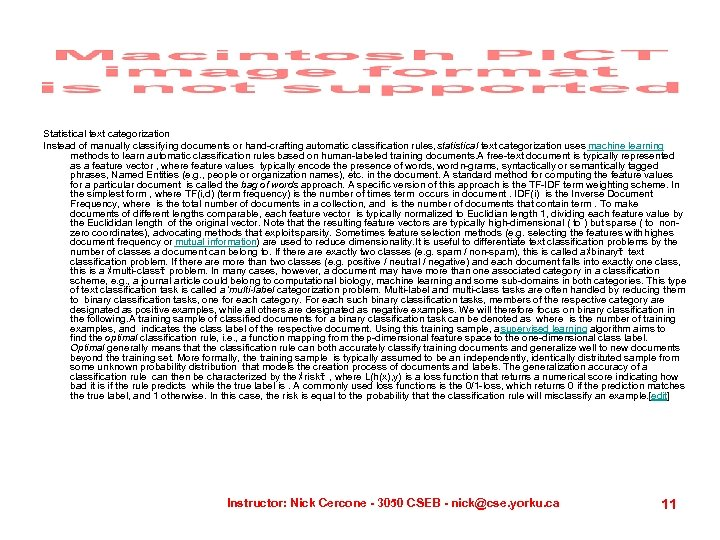Statistical text categorization Instead of manually classifying documents or hand-crafting automatic classification rules, statistical text categorization uses machine learning methods to learn automatic classification rules based on human-labeled training documents. A free-text document is typically represented as a feature vector , where feature values typically encode the presence of words, word n-grams, syntactically or semantically tagged phrases, Named Entities (e. g. , people or organization names), etc. in the document. A standard method for computing the feature values for a particular document is called the bag of words approach. A specific version of this approach is the TF-IDF term weighting scheme. In the simplest form , where TF(i, d) (term frequency) is the number of times term occurs in document. IDF(i) is the Inverse Document Frequency, where is the total number of documents in a collection, and is the number of documents that contain term. To make documents of different lengths comparable, each feature vector is typically normalized to Euclidian length 1, dividing each feature value by the Euclididan length of the original vector. Note that the resulting feature vectors are typically high-dimensional ( to ) but sparse ( to nonzero coordinates), advocating methods that exploit sparsity. Sometimes feature selection methods (e. g. selecting the features with highes document frequency or mutual information) are used to reduce dimensionality. It is useful to differentiate text classification problems by the number of classes a document can belong to. If there are exactly two classes (e. g. spam / non-spam), this is called aﾒbinaryﾓ text classification problem. If there are more than two classes (e. g. positive / neutral / negative) and each document falls into exactly one class, this is a ﾒmulti-classﾓ problem. In many cases, however, a document may have more than one associated category in a classification scheme, e. g. , a journal article could belong to computational biology, machine learning and some sub-domains in both categories. This type of text classification task is called a 'multi-label categorization problem. Multi-label and multi-class tasks are often handled by reducing them to binary classification tasks, one for each category. For each such binary classification tasks, members of the respective category are designated as positive examples, while all others are designated as negative examples. We will therefore focus on binary classification in the following. A training sample of classified documents for a binary classification task can be denoted as where is the number of training examples, and indicates the class label of the respective document. Using this training sample, a supervised learning algorithm aims to find the optimal classification rule, i. e. , a function mapping from the p-dimensional feature space to the one-dimensional class label. Optimal generally means that the classification rule can both accurately classify training documents and generalize well to new documents beyond the training set. More formally, the training sample is typically assumed to be an independently, identically distrib uted sample from some unknown probability distribution that models the creation process of documents and labels. The generalization accuracy of a classification rule can then be characterized by the ﾒriskﾓ , where L(h(x), y) is a loss function that returns a numerical score indicating how bad it is if the rule predicts while the true label is. A commonly used loss functions is the 0/1 -loss, which returns 0 if the prediction matches the true label, and 1 otherwise. In this case, the risk is equal to the probability that the classification rule will misclassify an example.  Instructor: Nick Cercone - 3050 CSEB - [email protected] yorku. ca 11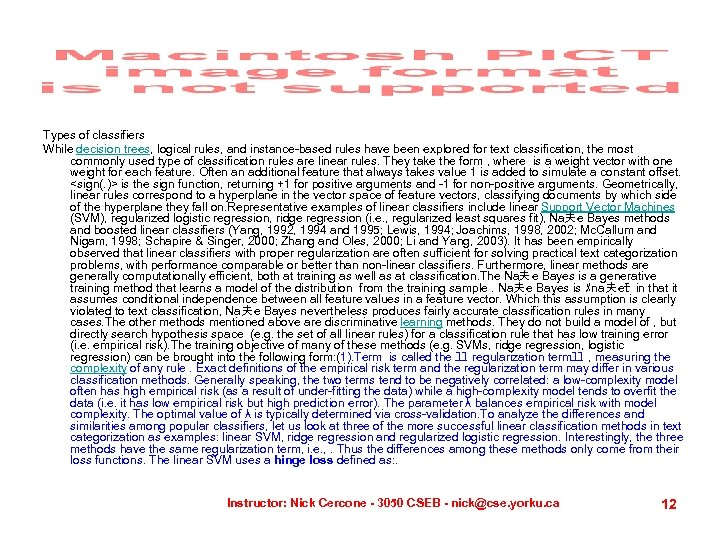Types of classifiers While decision trees, logical rules, and instance-based rules have been explored for text classification, the most commonly used type of classification rules are linear rules. They take the form , where is a weight vector with one weight for each feature. Often an additional feature that always takes value 1 is added to simulate a constant offset. is the sign function, returning +1 for positive arguments and -1 for non-positive arguments. Geometrically, linear rules correspond to a hyperplane in the vector space of feature vectors, classifying documents by which side of the hyperplane they fall on. Representative examples of linear classifiers include linear Support Vector Machines (SVM), regularized logistic regression, ridge regression (i. e. , regularized least squares fit), Na夫 e Bayes methods and boosted linear classifiers (Yang, 1992, 1994 and 1995; Lewis, 1994; Joachims, 1998, 2002; Mc. Callum and Nigam, 1998; Schapire & Singer, 2000; Zhang and Oles, 2000; Li and Yang, 2003). It has been empirically observed that linear classifiers with proper regularization are often sufficient for solving practical text categorization problems, with performance comparable or better than non-linear classifiers. Furthermore, linear methods are generally computationally efficient, both at training as well as at classification. The Na夫 e Bayes is a generative training method that learns a model of the distribution from the training sample. Na夫 e Bayes is ﾒna夫 eﾓ in that it assumes conditional independence between all feature values in a feature vector. Which this assumption is clearly violated to text classification, Na夫 e Bayes nevertheless produces fairly accurate classification rules in many cases. The other methods mentioned above are discriminative learning methods. They do not build a model of , but directly search hypothesis space (e. g. the set of all linear rules) for a classification rule that has low training error (i. e. empirical risk). The training objective of many of these methods (e. g. SVMs, ridge regression, logistic regression) can be brought into the following form: (1). Term is called the ﾕﾕ regularization termﾕﾕ , measuring the complexity of any rule. Exact definitions of the empirical risk term and the regularization term may differ in various classification methods. Generally speaking, the two terms tend to be negatively correlated: a low-complexity model often has high empirical risk (as a result of under-fitting the data) while a high-complexity model tends to overfit the data (i. e. it has low empirical risk but high prediction error). The parameter λ balances empirical risk with model complexity. The optimal value of λ is typically determined via cross-validation. To analyze the differences and similarities among popular classifiers, let us look at three of the more successful linear classification methods in text categorization as examples: linear SVM, ridge regression and regularized logistic regression. Interestingly, the three methods have the same regularization term, i. e. , . Thus the differences among these methods only come from their loss functions. The linear SVM uses a hinge loss defined as: . Instructor: Nick Cercone - 3050 CSEB - [email protected] yorku. ca 12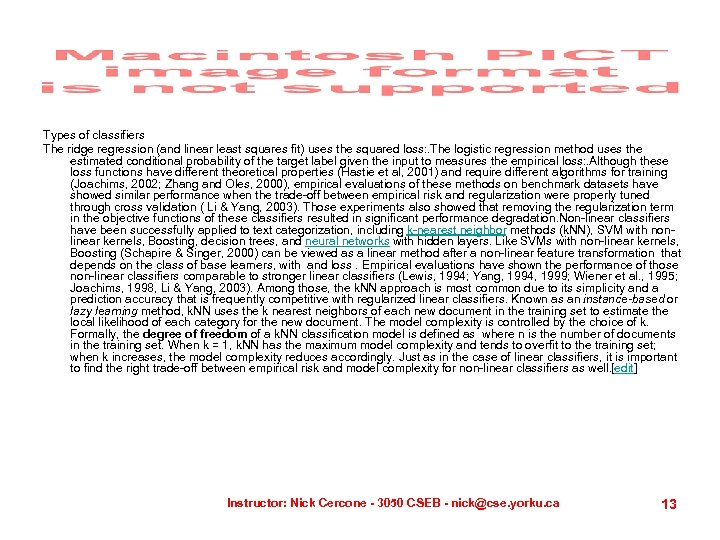Types of classifiers The ridge regression (and linear least squares fit) uses the squared loss: . The logistic regression method uses the estimated conditional probability of the target label given the input to measures the empirical loss: . Although these loss functions have different theoretical properties (Hastie et al, 2001) and require different algorithms for training (Joachims, 2002; Zhang and Oles, 2000), empirical evaluations of these methods on benchmark datasets have showed similar performance when the trade-off between empirical risk and regularization were properly tuned through cross validation ( Li & Yang, 2003). Those experiments also showed that removing the regularization term in the objective functions of these classifiers resulted in significant performance degradation. Non-linear classifiers have been successfully applied to text categorization, including k-nearest neighbor methods (k. NN), SVM with nonlinear kernels, Boosting, decision trees, and neural networks with hidden layers. Like SVMs with non-linear kernels, Boosting (Schapire & Singer, 2000) can be viewed as a linear method after a non-linear feature transformation that depends on the class of base learners, with and loss. Empirical evaluations have shown the performance of those non-linear classifiers comparable to stronger linear classifiers (Lewis, 1994; Yang, 1994, 1999; Wiener et al. , 1995; Joachims, 1998, Li & Yang, 2003). Among those, the k. NN approach is most common due to its simplicity and a prediction accuracy that is frequently competitive with regularized linear classifiers. Known as an instance-based or lazy learning method, k. NN uses the k nearest neighbors of each new document in the training set to estimate the local likelihood of each category for the new document. The model complexity is controlled by the choice of k. Formally, the degree of freedom of a k. NN classification model is defined as where n is the number of documents in the training set. When k = 1, k. NN has the maximum model complexity and tends to overfit to the training set; when k increases, the model complexity reduces accordingly. Just as in the case of linear classifiers, it is important to find the right trade-off between empirical risk and model complexity for non-linear classifiers as well.  Instructor: Nick Cercone - 3050 CSEB - [email protected] yorku. ca 13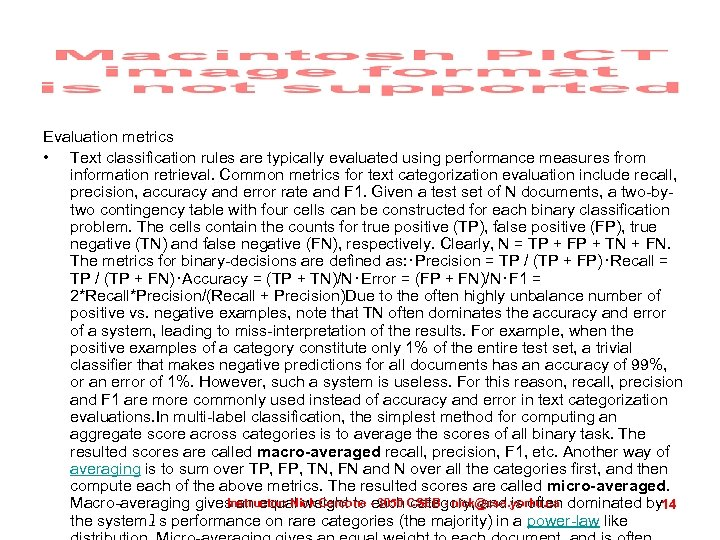Evaluation metrics • Text classification rules are typically evaluated using performance measures from information retrieval. Common metrics for text categorization evaluation include recall, precision, accuracy and error rate and F 1. Given a test set of N documents, a two-bytwo contingency table with four cells can be constructed for each binary classification problem. The cells contain the counts for true positive (TP), false positive (FP), true negative (TN) and false negative (FN), respectively. Clearly, N = TP + FP + TN + FN. The metrics for binary-decisions are defined as: ･Precision = TP / (TP + FP)･Recall = TP / (TP + FN)･Accuracy = (TP + TN)/N･Error = (FP + FN)/N･F 1 = 2*Recall*Precision/(Recall + Precision)Due to the often highly unbalance number of positive vs. negative examples, note that TN often dominates the accuracy and error of a system, leading to miss-interpretation of the results. For example, when the positive examples of a category constitute only 1% of the entire test set, a trivial classifier that makes negative predictions for all documents has an accuracy of 99%, or an error of 1%. However, such a system is useless. For this reason, recall, precision and F 1 are more commonly used instead of accuracy and error in text categorization evaluations. In multi-label classification, the simplest method for computing an aggregate score across categories is to average the scores of all binary task. The resulted scores are called macro-averaged recall, precision, F 1, etc. Another way of averaging is to sum over TP, FP, TN, FN and N over all the categories first, and then compute each of the above metrics. The resulted scores are called micro-averaged. Instructor: Nick Cercone each category, and is often Macro-averaging gives an equal weight to - 3050 CSEB - [email protected] yorku. ca dominated by 14 the systemﾕ s performance on rare categories (the majority) in a power-law like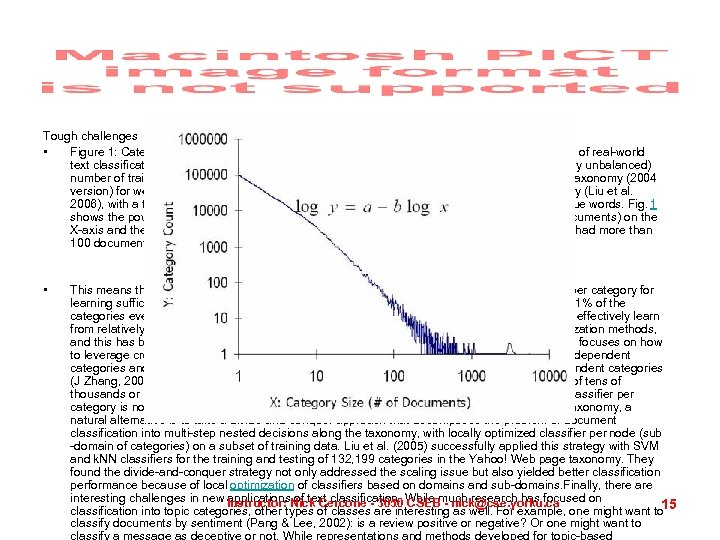Tough challenges • Figure 1: Category sizes in Yahoo! Web-page Taxonomy (2004) exhibits a power law. The scale of real-world text classification applications, both in terms of the number of classes as well as the (often highly unbalanced) number of training examples, poses interesting research challenges. For example, the Yahoo! taxonomy (2004 version) for web page classification contains nearly 300, 000 categories over a 16 -level hierarchy (Liu et al. 2006), with a total of approximately 800, 000 documents and a vocabulary of over 4 million unique words. Fig. 1 shows the power-law correspondence between the category size (in terms of the number of documents) on the X-axis and the number of same-sized categories on the Y-axis. Less than 1% of the categories had more than 100 documents per category at the time the taxonomy was crawled in 2004. • This means that if a statistical classifier requires approximately 100 positive training examples per category for learning sufficiently accurate models, then we can only solve the classification problem for only 1% of the categories even if we use the entire set of 800, 000 pages as training examples. Clearly, how to effectively learn from relatively sparse training examples is therefore crucial for the true success of text categorization methods, and this has been a challenging research topic. Recent work in multi-task learning, for example, focuses on how to leverage cross-category dependencies, how to ﾒborrowﾓ training examples among mutually dependent categories and how to discover latent structures in a functional space for joint modeling of dependent categories (J Zhang, 2006; T Zhang, 2005, 2007). When the number of categories reaches the magnitude of tens of thousands or higher, the conventional approach of using all the documents to train a two-way classifier per category is no longer computationally feasible. If the categories are arranged in a hierarchical taxonomy, a natural alternative is to take a divide-and-conquer approach that decomposes the problem of document classification into multi-step nested decisions along the taxonomy, with locally optimized classifier per node (sub -domain of categories) on a subset of training data. Liu et al. (2005) successfully applied this strategy with SVM and k. NN classifiers for the training and testing of 132, 199 categories in the Yahoo! Web page taxonomy. They found the divide-and-conquer strategy not only addressed the scaling issue but also yielded better classification performance because of local optimization of classifiers based on domains and sub-domains. Finally, there are interesting challenges in new Instructor: Nick Cercone - 3050 While much research has focused on applications of text classification. CSEB - [email protected] yorku. ca 15 classification into topic categories, other types of classes are interesting as well. For example, one might want to classify documents by sentiment (Pang & Lee, 2002): is a review positive or negative? Or one might want to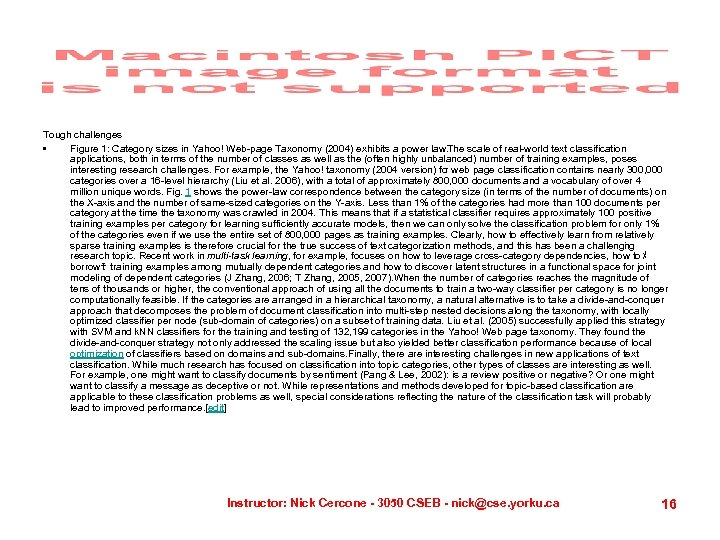Tough challenges • Figure 1: Category sizes in Yahoo! Web-page Taxonomy (2004) exhibits a power law. The scale of real-world text classification applications, both in terms of the number of classes as well as the (often highly unbalanced) number of training examples, poses interesting research challenges. For example, the Yahoo! taxonomy (2004 version) fo web page classification contains nearly 300, 000 r categories over a 16 -level hierarchy (Liu et al. 2006), with a total of approximately 800, 000 documents and a vocabulary of over 4 million unique words. Fig. 1 shows the power-law correspondence between the category size (in terms of the number of documents) on the X-axis and the number of same-sized categories on the Y-axis. Less than 1% of the categories had more than 100 documents per category at the time the taxonomy was crawled in 2004. This means that if a statistical classifier requires approximately 100 positive training examples per category for learning sufficiently accurate models, then we can only solve the classification problem for only 1% of the categories even if we use the entire set of 800, 000 pages as training examples. Clearly, how to effectively learn from relatively sparse training examples is therefore crucial for the true success of text categorization methods, and this has been a challengi g n research topic. Recent work in multi-task learning, for example, focuses on how to leverage cross-category dependencies, how to ﾒ borrowﾓ training examples among mutually dependent categories and how to discover latent structures in a functional space for joint modeling of dependent categories (J Zhang, 2006; T Zhang, 2005, 2007). When the number of categories reaches the magnitude of tens of thousands or higher, the conventional approach of using all the documents to train a two-way classifier per category is no longer computationally feasible. If the categories are arranged in a hierarchical taxonomy, a natural alternative is to take a divide-and-conquer approach that decomposes the problem of document classification into multi-step nested decisions along the taxonomy, with locally optimized classifier per node (sub-domain of categories) on a subset of training data. Liu et al. (2005) successfully applied this strategy with SVM and k. NN classifiers for the training and testing of 132, 199 categories in the Yahoo! Web page taxonomy. They found the divide-and-conquer strategy not only addressed the scaling issue but also yielded better classification performance because of local optimization of classifiers based on domains and sub-domains. Finally, there are interesting challenges in new applications of text classification. While much research has focused on classification into topic categories, other types of classes are interesting as well. For example, one might want to classify documents by sentiment (Pang & Lee, 2002): is a review positive or negative? Or one might want to classify a message as deceptive or not. While representations and methods developed for topic-based classification are applicable to these classification problems as well, special considerations reflecting the nature of the classification task will probably lead to improved performance.  Instructor: Nick Cercone - 3050 CSEB - [email protected] yorku. ca 16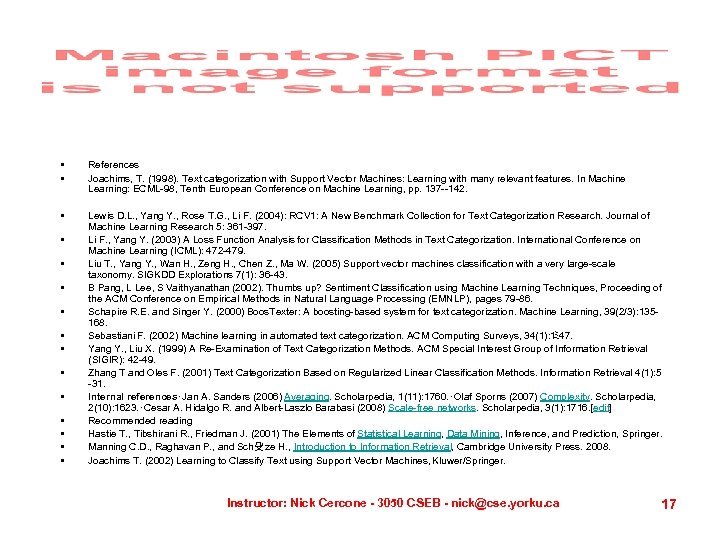• • References Joachims, T. (1998). Text categorization with Support Vector Machines: Learning with many relevant features. In Machine Learning: ECML-98, Tenth European Conference on Machine Learning, pp. 137 --142. • Lewis D. L. , Yang Y. , Rose T. G. , Li F. (2004): RCV 1: A New Benchmark Collection for Text Categorization Research. Journal of Machine Learning Research 5: 361 -397. Li F. , Yang Y. (2003) A Loss Function Analysis for Classification Methods in Text Categorization. International Conference on Machine Learning (ICML): 472 -479. Liu T. , Yang Y. , Wan H. , Zeng H. , Chen Z. , Ma W. (2005) Support vector machines classification with a very large-scale taxonomy. SIGKDD Explorations 7(1): 36 -43. B Pang, L Lee, S Vaithyanathan (2002). Thumbs up? Sentiment Classification using Machine Learning Techniques, Proceeding of the ACM Conference on Empirical Methods in Natural Language Processing (EMNLP), pages 79 -86. Schapire R. E. and Singer Y. (2000) Boos. Texter: A boosting-based system for text categorization. Machine Learning, 39(2/3): 135168. Sebastiani F. (2002) Machine learning in automated text categorization. ACM Computing Surveys, 34(1): 1 ﾐ 47. Yang Y. , Liu X. (1999) A Re-Examination of Text Categorization Methods. ACM Special Interest Group of Information Retrieval (SIGIR): 42 -49. Zhang T and Oles F. (2001) Text Categorization Based on Regularized Linear Classification Methods. Information Retrieval 4(1): 5 -31. Internal references･Jan A. Sanders (2006) Averaging. Scholarpedia, 1(11): 1760. ･Olaf Sporns (2007) Complexity. Scholarpedia, 2(10): 1623. ･Cesar A. Hidalgo R. and Albert-Laszlo Barabasi (2008) Scale-free networks. Scholarpedia, 3(1): 1716.  Recommended reading Hastie T. , Tibshirani R. , Friedman J. (2001) The Elements of Statistical Learning, Data Mining, Inference, and Prediction, Springer. Manning C. D. , Raghavan P. , and Sch殳ze H. , Introduction to Information Retrieval, Cambridge University Press. 2008. Joachims T. (2002) Learning to Classify Text using Support Vector Machines, Kluwer/Springer. • • • Instructor: Nick Cercone - 3050 CSEB - [email protected] yorku. ca 17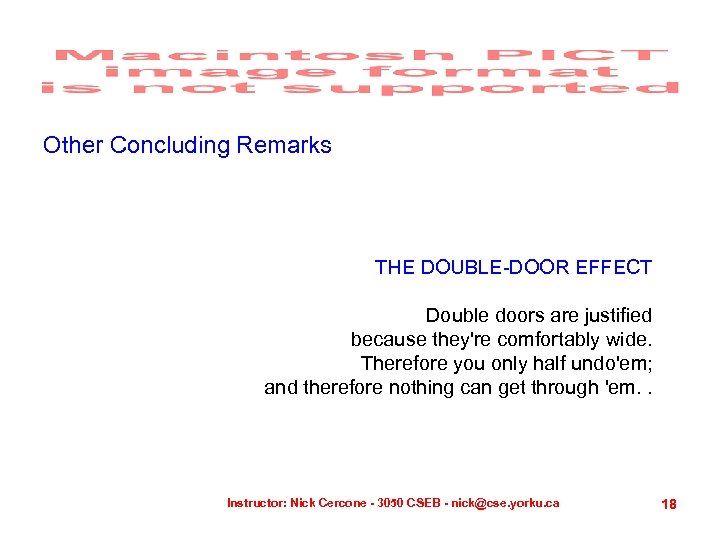Other Concluding Remarks THE DOUBLE-DOOR EFFECT Double doors are justified because they're comfortably wide. Therefore you only half undo'em; and therefore nothing can get through 'em. . Instructor: Nick Cercone - 3050 CSEB - [email protected] yorku. ca 18